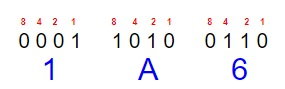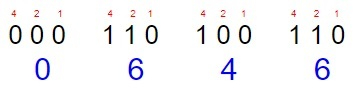# Program to Convert Hexadecimal to Octal in C program

We are given a Hexadecimal number as string; the task is to convert it to the Octal. To convert a hexadecimal number to octal, we have to −

• Find the binary equivalent to the hexadecimal number.
• Convert the binary number to Octal.

Hexadecimal numbers are the numbers which are of base of 16 the numbers vary from 0-9 and from 10 onwards the numbers are represented as A which represents 10, B for 11, C for 12, D for 13, E for 14, and F for 15.

To convert hexadecimal number into binary number every number is converted into its binary equivalent of 4 bits and after that these numbers are combined to form one corresponding binary number.

## What are octal numbers

Octal numbers in computers are represented with base 8, which are form 0-7 octal numbers are made by grouping of three binary numbers or three binary digits.

## What we have to do

Like we have a hexadecimal number 1A6 so it means 1, 10 and 6 now for hexadecimal to octal first we have to find the binary equivalent of a hexadecimal number i.e.,So, the binary of 1A6 = 0001 1010 0110

Now after finding the binary of the hexadecimal number now the next task is to find the octal of the binary number.

Before that we will group the binary number in three. After grouping in 3 we will get 000 110 100 110

Whose octal representation will be −So the octal representation of hexadecimal number 1A6 is − 646

## Example

Input: 1A6
Output: Octal Value = 646
Explanation:

Input: 1AA
Output: 652

Approach we will be using to solve the given problem −

• Taking the input and store it as a string.
• Convert the hexadecimal number or expression to binary by following the below approach −
• Check for all the 16 cases of hexadecimal by adding their respective binary representation.
• Return the result.
• Convert the binary number to octal number follow the following steps −
• Take the 3 places by comparing all the possible cases of binary number to octal.
• Set the value of octal = (val * place) + octal;
• Divide binary number by 1000
• place *= 10
• Return the result.

## Algorithm

Start
Step 1-> In function long long int hexa_binary(char hex[])
Declare variables binary, place
Declare and initialize i = 0, rem, val
Initialize t n = strlen(hex)
Initialize binary = 0ll and place = 0ll
Loop For i = 0 and hex[i] != '\0' and i++ {
binary = binary * place;
switch (hex[i]) {
case '0':
binary += 0
case '1':
binary += 1
case '2':
binary += 10
case '3':
binary += 11
case '4':
binary += 100
case '5':
binary += 101
case '6':
binary += 110
case '7':
binary += 111
case '8':
binary += 1000
case '9':
binary += 1001
case 'a':
case 'A':
binary += 1010
case 'b':
case 'B':
binary += 1011
case 'c':
case 'C':
binary += 1100
case 'd':
case 'D':
binary += 1101;
break;
case 'e':
case 'E':
binary += 1110;
break;
case 'f':
case 'F':
binary += 1111;
break;
default:
}
place = 10000;
}
return binary;
}
long long int binary_oct(long long binary) {
long long int octal, place;
int i = 0, rem, val;
octal = 0ll;
place = 0ll;
place = 1;
while (binary > 0) {
rem = binary % 1000;
switch (rem) {
case 0:
val = 0;
break;
case 1:
val = 1;
break;
case 10:
val = 2;
break;
case 11:
val = 3;
break;
case 100:
val = 4;
break;
case 101:
val = 5;
break;
case 110:
val = 6;
break;
case 111:
val = 7;
break;
}
octal = (val * place) + octal;
binary /= 1000;
place *= 10;
}
return octal;
}
long long int hexa_oct(char hex[]) {
long long int octal, binary;
binary = hexa_binary(hex);
// convert Binary to Octal
octal = binary_oct(binary);
return octal;
}
int main() {
char hex = "1a99";
printf("Octal Value = %lld", hexa_oct(hex));
return 0;
}

## Example

#include <stdio.h>
#include <string.h>
#include <math.h>
//To convert hex to binary first
long long int hexa_binary(char hex[]) {
long long int binary, place;
int i = 0, rem, val;
int n = strlen(hex);
binary = 0ll;
place = 0ll;
for (i = 0; hex[i] != '\0'; i++) {
binary = binary * place;
switch (hex[i]) {
case '0':
binary += 0;
break;
case '1':
binary += 1;
break;
case '2':
binary += 10;
break;
case '3':
binary += 11;
break;
case '4':
binary += 100;
break;
case '5':
binary += 101;
break;
case '6':
binary += 110;
break;
case '7':
binary += 111;
break;
case '8':
binary += 1000;
break;
case '9':
binary += 1001;
break;
case 'a':
case 'A':
binary += 1010;
break;
case 'b':
case 'B':
binary += 1011;
break;
case 'c':
case 'C':
binary += 1100;
break;
case 'd':
case 'D':
binary += 1101;
break;
case 'e':
case 'E':
binary += 1110;
break;
case 'f':
case 'F':
binary += 1111;
break;
default:
}
place = 10000;
}
return binary;
}
//To convert binary to octal
long long int binary_oct(long long binary) {
long long int octal, place;
int i = 0, rem, val;
octal = 0ll;
place = 0ll;
place = 1;
// giving all binary numbers for octal conversion
while (binary > 0) {
rem = binary % 1000;
switch (rem) {
case 0:
val = 0;
break;
case 1:
val = 1;
break;
case 10:
val = 2;
break;
case 11:
val = 3;
break;
case 100:
val = 4;
break;
case 101:
val = 5;
break;
case 110:
val = 6;
break;
case 111:
val = 7;
break;
}
octal = (val * place) + octal;
binary /= 1000;
place *= 10;
}
return octal;
}
// to convert the hexadecimal number to octal
long long int hexa_oct(char hex[]) {
long long int octal, binary;
binary = hexa_binary(hex);
// convert Binary to Octal
octal = binary_oct(binary);
return octal;
}
//main function
int main() {
char hex = "5CD";
printf("Octal Value = %lld", hexa_oct(hex));
return 0;
}

## Output

Octal Value = 2715